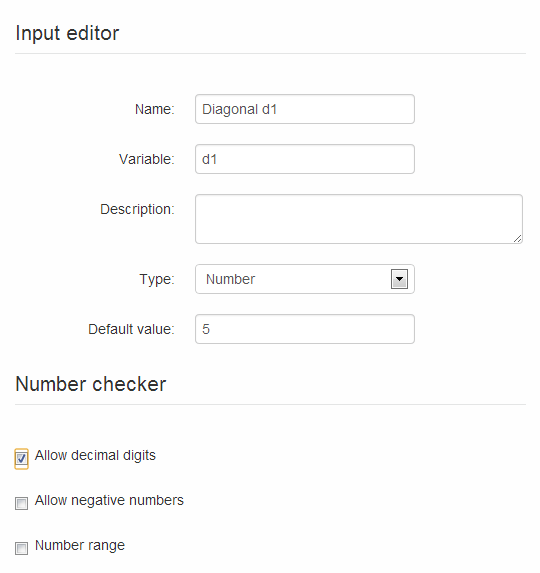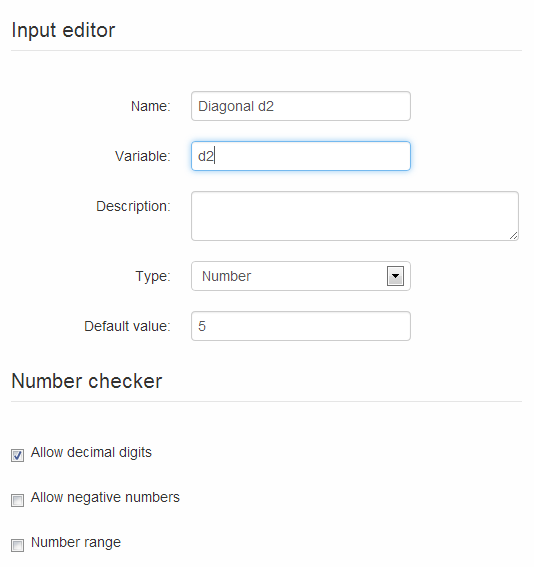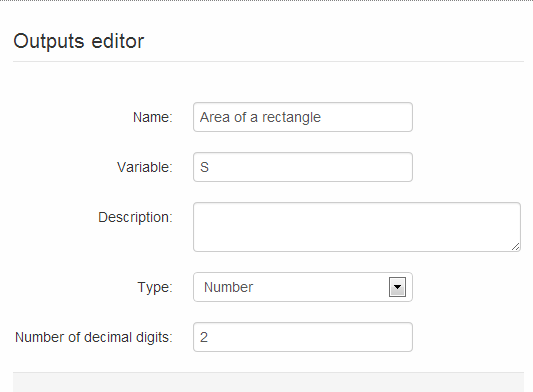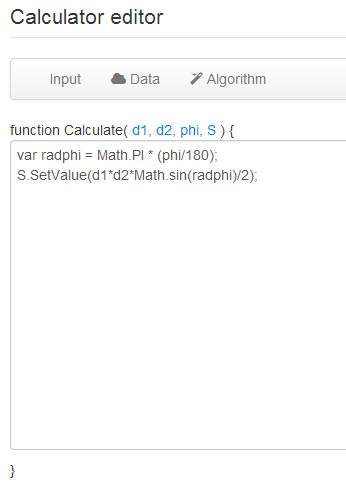homechevron_rightStudychevron_rightMathchevron_rightGeometry

# Area of a convex quadrilateral. Example of creating a calculator.

This online calculator calculates area of a convex quadrilateral given diagonal values and value of angle between them. Article also describes the process of creating a calculator.

### This page exists due to the efforts of the following people:

#### Anton

Visitors keep asking about calculator which would compute area of a convex quadrilateral given it's sides. This is mathematically impossible. However, you can find area of a convex quadrilateral, given quadrilateral diagonal values and value of angle between them.

Formula:

The calculator for the formula is below. After calculator I describe the process of creating a calculator using this site's engine.#### Area of a convex quadrilateral

Digits after the decimal point: 2

This site is an engine to create online calculators. All you need to create such calculators is to be registered user and understand basic Javascript syntax.

To create a calculator, you first should log in into site. Then, in "Personal section" you should choose "Create" -> "Calculator" menu item.

You will get this formThe calculator, basically, is the thing, which receives user input and convert it to output values. So, for our calculator, we need three input parameters: two diagonals and angle. We have to describe them for the engine.

Choose "Input" -> "Add new input parameter" in menu. You will get input parameter editor, which should be filled as on the picture.Repeat for second parameterFor the third parameter use range feature and limit allowed values with range from 0 to 180 degrees.Note that each time you change the parameters, signature of function "Calculate" is changed to reflect those.

Now you have to describe output parameter. Choose "Output" -> "New output parameter" and fill the form as below.Now we have described all ins and outs, so the only thing we need is to make conversion of inputs to outputs inside "Calculate" function. Here you will need Javascript.

Note that input parameters can be used directly, however, output parameter is an object, and the only way to assign value to it, is to use it's special method "SetValue".

Code which calculates area:First line: note the conversion of degrees to radians and usage of Javascript built-in Math object.
Second line: note the usage of SetValue to assign calculation result.

Now you can press "Preview". This button allows you to preview and test the calculations and also saves the calculator source on site.Now you can publish calculator using button "Publish". After publishing, your calculator will get constant URL on site and will be available for everyone.

The final step is to create article which describes the calculator. Article lets you educate visitors about your calculator, its purpose and details of calculation, and almost always user friendly. To create an article you should choose "Create" -> "Article" in "Personal Section". Then you write some text and insert the calculator into your article, using "Calculator" button on toolbar.

URL copied to clipboardPLANETCALC, Area of a convex quadrilateral. Example of creating a calculator.